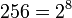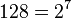# Dihedral group:D256

View a complete list of particular groups (this is a very huge list!)[SHOW MORE]

## Definition

This group is defined as the dihedral group of order$256 = 2^8$ and degree$128 = 2^7$.

## Arithmetic functions

Want to compare and contrast arithmetic function values with other groups of the same order? Check out groups of order 256#Arithmetic functions
Function Value Similar groups Explanation for function value GAP verification (set G := DihedralGroup(256);) -- See more at #GAP implementation
underlying prime of p-group 2
order (number of elements, equivalently, cardinality or size of underlying set) 256 groups with same order Order(G); using Order.
prime-base logarithm of order 8 groups with same prime-base logarithm of order
max-length of a group 8 max-length of a group equals prime-base logarithm of order for group of prime power order
chief length 8 chief length equals prime-base logarithm of order for group of prime power order
composition length 8 composition length equals prime-base logarithm of order for group of prime power order
exponent of a group 128 groups with same order and exponent of a group | groups with same exponent of a group cyclic subgroup of order 128. Exponent(G); using Exponent.
prime-base logarithm of exponent 7 groups with same order and prime-base logarithm of exponent | groups with same prime-base logarithm of order and prime-base logarithm of exponent | groups with same prime-base logarithm of exponent
nilpotency class 7 groups with same order and nilpotency class | groups with same prime-base logarithm of order and nilpotency class | groups with same nilpotency class NilpotencyClassOfGroup(G); using NilpotencyClassOfGroup.
derived length 2 groups with same order and derived length | groups with same prime-base logarithm of order and derived length | groups with same derived length the derived subgroup is contained in the cyclic subgroup and is hence abelian DerivedLength(G); using DerivedLength.
Frattini length 7 groups with same order and Frattini length | groups with same prime-base logarithm of order and Frattini length | groups with same Frattini length The first Frattini subgroup is cyclic generated by$a^2$, each subsequent Frattini subgroup moves to the set of squares of its predecessor. FrattiniLength(G); using FrattiniLength.
minimum size of generating set 2 groups with same order and minimum size of generating set | groups with same prime-base logarithm of order and minimum size of generating set | groups with same minimum size of generating set Rank(G); using Rank.
subgroup rank of a group 2 groups with same order and subgroup rank of a group | groups with same prime-base logarithm of order and subgroup rank of a group | groups with same subgroup rank of a group All proper subgroups are cyclic, dihedral, or Klein four-groups. SubgroupRank(G); using SubgroupRank.
rank of a p-group 2 groups with same order and rank of a p-group | groups with same prime-base logarithm of order and rank of a p-group | groups with same rank of a p-group there exist Klein four-subgroups. RankAsPGroup(G); using RankAsPGroup.
normal rank of a p-group 1 groups with same order and normal rank of a p-group | groups with same prime-base logarithm of order and normal rank of a p-group | groups with same normal rank of a p-group all abelian normal subgroups are cyclic. NormalRank(G); using NormalRank.
characteristic rank of a p-group 1 groups with same order and characteristic rank of a p-group | groups with same prime-base logarithm of order and characteristic rank of a p-group | groups with same characteristic rank of a p-group All abelian characteristic subgroups are cyclic. CharacteristicRank(G); using CharacteristicRank.

## GAP implementation

### Group ID

This finite group has order 256 and has ID 539 among the groups of order 256 in GAP's SmallGroup library. For context, there are groups of order 256. It can thus be defined using GAP's SmallGroup function as:

SmallGroup(256,539)

For instance, we can use the following assignment in GAP to create the group and name it$G$:

gap> G := SmallGroup(256,539);

Conversely, to check whether a given group$G$ is in fact the group we want, we can use GAP's IdGroup function:

IdGroup(G) = [256,539]

or just do:

IdGroup(G)

to have GAP output the group ID, that we can then compare to what we want.